### Home > CALC > Chapter 5 > Lesson 5.3.1 > Problem5-113

5-113.
1. FUNCTIONS FROM TABLES, Part Two

2. Find a function, f(x), that fits the table shown below. Homework Help ✎

3.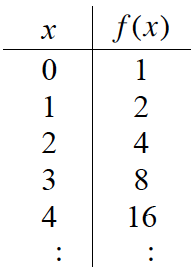1. What kind of function does this appear to be? Find a possible equation.

2. How does the function change? Find the difference between the terms in f(x), what kind of function is represented by these terms?

3. Find f(5). Are you sure you are correct?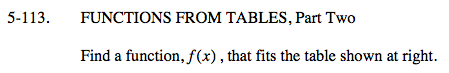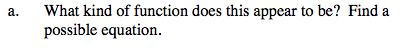Is it linear? Quadratic? Cubic? Exponential? Something else?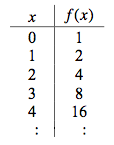Δy

2 − 1 = 1
4 − 2 = 2
8 − 4 = 4
16 − 8 = 8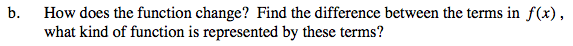What pattern do you see among the finite differences (Δy) pattern?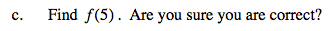Also determine the next finite difference term.